GFG App
Open AppBrowser
Continue

# Python | Pandas dataframe.reindex_like()

Python is a great language for doing data analysis, primarily because of the fantastic ecosystem of data-centric python packages. Pandas is one of those packages and makes importing and analyzing data much easier.

Pandas` dataframe.reindex_like()` function return an object with matching indices to myself. Any non-matching indexes are filled with `NaN` values.

Syntax:
Syntax : DataFrame.reindex_like(other, method=None, copy=True, limit=None, tolerance=None)

Parameters :
other : Object
method : string or None
copy : boolean, default True
limit : Maximum number of consecutive labels to fill for inexact matches.
tolerance : Maximum distance between labels of the other object and this object for inexact matches. Can be list-like.

Returns : reindexed : same as input

Example #1: Use `reindex_like()` function to find the matching indexes between the given two dataframes.

Note : We can fill in the missing values by using any of the fill methods (ex. ‘ffill’, ‘bfill’).

 `# importing pandas as pd ` `import` `pandas as pd ` ` `  `# Creating the first dataframe  ` `df1 ``=` `pd.DataFrame({``"A"``:[``1``, ``5``, ``3``, ``4``, ``2``], ` `                    ``"B"``:[``3``, ``2``, ``4``, ``3``, ``4``], ` `                    ``"C"``:[``2``, ``2``, ``7``, ``3``, ``4``], ` `                    ``"D"``:[``4``, ``3``, ``6``, ``12``, ``7``]}, ` `                    ``index ``=``[``"A1"``, ``"A2"``, ``"A3"``, ``"A4"``, ``"A5"``]) ` ` `  `# Creating the second dataframe ` `df2 ``=` `pd.DataFrame({``"A"``:[``10``, ``11``, ``7``, ``8``, ``5``],  ` `                    ``"B"``:[``21``, ``5``, ``32``, ``4``, ``6``], ` `                    ``"C"``:[``11``, ``21``, ``23``, ``7``, ``9``],  ` `                    ``"D"``:[``1``, ``5``, ``3``, ``8``, ``6``]},  ` `                     ``index ``=``[``"A1"``, ``"A3"``, ``"A4"``, ``"A7"``, ``"A8"``]) ` ` `  `# Print the first dataframe ` `df1 ` ` `  `# Print the second dataframe ` `df2 `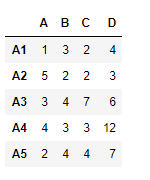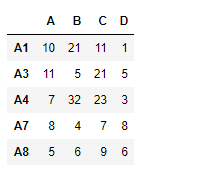Let’s use the `dataframe.reindex_like()` function to find the matching indexes.

 `# find matching indexes ` `df1.reindex_like(df2) `

Output :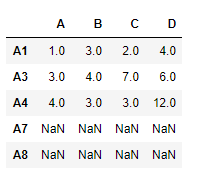Notice the output, unmatched indexes are populated with `NaN` values, we can fill in the missing values using ‘ffill’ method.

 `# filling the missing values using ffill method ` `df1.reindex_like(df2, method ``=``'ffill'``) `

Output :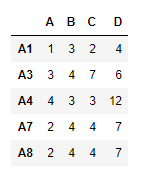Notice in the output, the new indexes has been populated using the “A5” row.

Example #2: Use `reindex_like()` function to match the indexes of two dataframes with limit on filling the missing values.

 `# importing pandas as pd ` `import` `pandas as pd ` ` `  `# Creating the first dataframe  ` `df1 ``=` `pd.DataFrame({``"A"``:[``1``, ``5``, ``3``, ``4``, ``2``], ` `                    ``"B"``:[``3``, ``2``, ``4``, ``3``, ``4``], ` `                    ``"C"``:[``2``, ``2``, ``7``, ``3``, ``4``], ` `                    ``"D"``:[``4``, ``3``, ``6``, ``12``, ``7``]}, ` `                    ``index ``=``[``"A1"``, ``"A2"``, ``"A3"``, ``"A4"``, ``"A5"``]) ` ` `  `# Creating the second dataframe ` `df2 ``=` `pd.DataFrame({``"A"``:[``10``, ``11``, ``7``, ``8``, ``5``], ` `                    ``"B"``:[``21``, ``5``, ``32``, ``4``, ``6``], ` `                    ``"F"``:[``11``, ``21``, ``23``, ``7``, ``9``], ` `                    ``"K"``:[``1``, ``5``, ``3``, ``8``, ``6``]}, ` `                    ``index ``=``[``"A1"``, ``"A2"``, ``"A3"``, ``"A4"``, ``"A7"``]) ` ` `  `# matching the indexes ` `df1.reindex_like(df2) `

Output :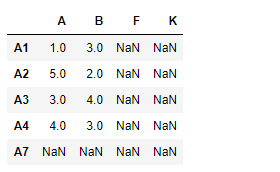Notice the output, unmatched indexes are populated with `NaN` values, we can fill in the missing values using ‘ffill’ method. we also limit the number of consecutive unmatched indexes that could be filled using the limit parameter.

 `# match the indexes ` `# fill the unmatched index using 'ffill' method ` `# maximum consecutive unmatched indexes to be filled is 1 ` ` `  `df.reindex_like(df1, method ``=``'ffill'``, limit ``=` `1``) `

Output :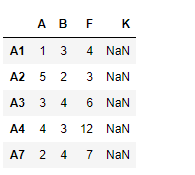My Personal Notes arrow_drop_up